Author: Oscar Cronquist Article last updated on December 21, 2022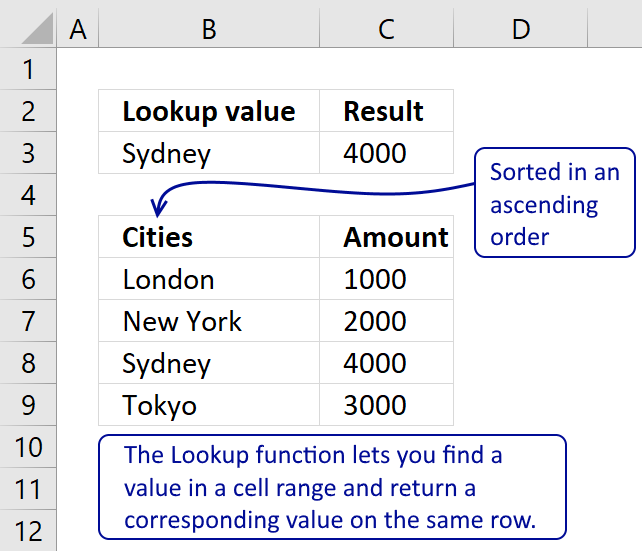The Lookup function lets you find a value in a cell range and return a corresponding value on the same row.

## 1. LOOKUP Function Syntax

LOOKUP(lookup_value, lookup_vector, [result_vector])

If the LOOKUP function can't find the lookup_value, the function matches the largest value in lookup_vector that is less than or equal to lookup_value.

If lookup_value is smaller than the smallest value in lookup_vector, LOOKUP returns the #N/A error value.

## 2. LOOKUP Function Arguments

 lookup_value Required. The lookup value can be a number, text or a logical value. It can also be a cell reference. This argument can also handle multiple values but you need to enter it as an array function to make it work, there is an example later in this post. lookup_vector Required. This argument must be a single column or single row cell range. result_vector Required. The result vector is optional. If omitted the function returns the lookup value, if found. This argument must be a single column or single row cell range and have the same size as the lookup vector.

## 3. Be careful with the LOOKUP function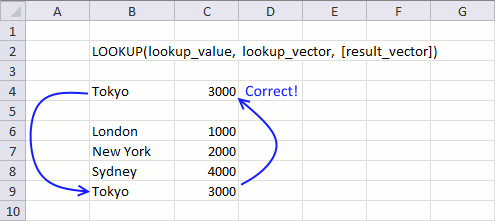The values in lookup_vector must be sorted in ascending order or from A to Z! I recommend the VLOOKUP function, INDEX and MATCH functions, or XLOOKUP function.

The values in lookup_vector must be sorted ascending or from A to Z but that is not all. You may think you get an error if the lookup value is not found but that is not always the case.

Only if the lookup_value is smaller than the smallest value in lookup_vector, LOOKUP returns #N/A. See cell B4 and C4 in this animated picture.

So why does "Rome" return 2000? LOOKUP function can't find the lookup_value so it matches the largest value in lookup_vector that is less than or equal to lookup_value.

If Rome would have been in this sorted list, it would be between New York and Sydney. New York is less (alphabetically) than Rome but greater than London. The corresponding value to New York is returned, that value is 2000.

Anchorage is smaller than the smallest value "London" (alphabetically) and Lookup returns #N/A.

You really need to know what you are doing if you are going to use this function. I do recommend using this function with numerical ranges, see next example.

## 4. Find the largest value in lookup_vector that is less than or equal to lookup_value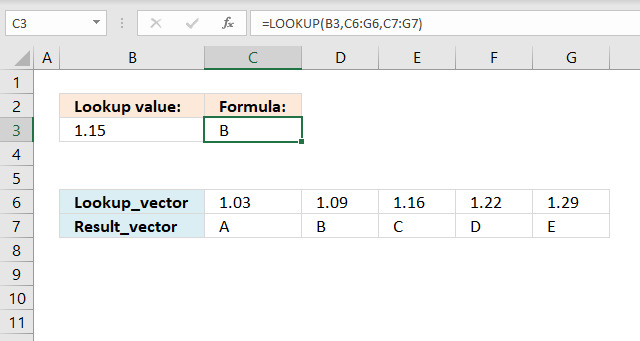This example uses a numerical value as a lookup value specified in cell B3. If an exact match is not found the closest value is returned as long as it is smaller than the lookup value.

Formula in cell C3:

=LOOKUP(B3, C6:G6, C7:G7)

becomes

LOOKUP(1.15, {1.03,1.09,1.16,1.22,1.29}, {"A","B","C","D","E"})

and returns "B" in cell C3..

The largest value that is smaller or equal to 1.15 is 1.09. The corresponding value to 1.09 is B.

## 5. How to use multiple values in the LOOKUP function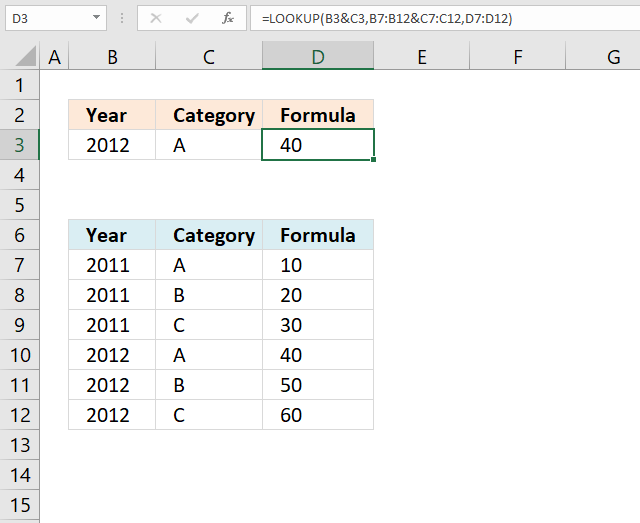You can concatenate values and search two columns, use the ampersand character "&" to concatenate values in an argument.

Note that the table is sorted by column B and then by column C. This is required in order to get reliable results.

Formula in cell D3:

=LOOKUP(B3&C3, B7:B12&C7:C12, D7:D12)

### Explaining formula in cell D2

LOOKUP(lookup_value, lookup_vector, [result_vector])

#### Step 1 - Concatenate lookup_value

B3&C3

becomes

2012&"A"

and returns "2012A".

#### Step 2 - Concatenate lookup_vector

B7:B12&C7:C12

becomes

{2011; 2011; 2011; 2012; 2012; 2012}&{"A"; "B"; "C"; "A"; "B"; "C"}

and returns

{"2011A"; "2011B"; "2011C"; "2012A"; "2012B"; "2012C"}

#### Step 3 - Evaluate LOOKUP function

LOOKUP(B3&C3, B7:B12&C7:C12, D7:D12)

becomes

LOOKUP("2012A", {"2011A"; "2011B"; "2011C"; "2012A"; "2012B"; "2012C"}, {10;20;30;40;50;60})

and returns 40 in cell D3.

## 6. How to make the LOOKUP function work with multiple worksheets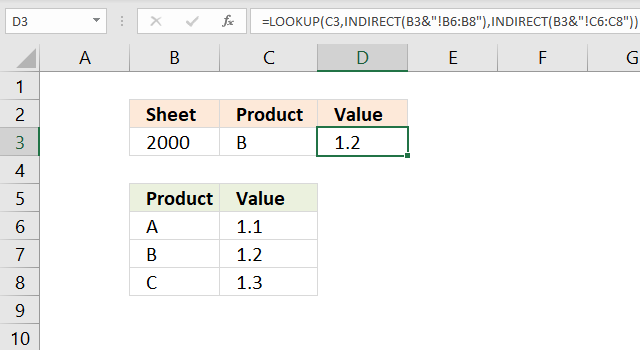This formula allows you to specify a sheet name and a lookup value. The INDIRECT function returns the cell reference depending on the value in cell B3.

Formula in cell D3:

=LOOKUP(C3, INDIRECT(B3&"!B6:B8"), INDIRECT(B3&"!C6:C8"))

### Explaining formula in cell D2

Note that the table is sorted by column B and then by column C. This is required in order to use the LOOKUP function.

LOOKUP(lookup_value, lookup_vector, [result_vector])

#### Step 1 - Create cell reference to another worksheet (lookup_vector)

The INDIRECT function is able to create a working cell reference containing a worksheet name specified in cell B3.

Change the worksheet name and the LOOKUP function changes immediately to the given worksheet name.

INDIRECT(B3&"!B6:B8")

becomes

INDIRECT("2000!B6:B8")

and returns "2000!B6:B8". Note that there is an exclamation mark between the worksheet name and the cell reference.

#### Step 2 - Create cell reference to another worksheet ([result_vector])

INDIRECT(B3&"!C6:C8")

becomes

INDIRECT("2000!C6:C8")

and returns "2000!C6:C8".

#### Step 3 - Evaluate LOOKUP function

LOOKUP(C3,INDIRECT(B3&"!B6:B8"),INDIRECT(B3&"!C6:C8"))

becomes

LOOKUP("B", 2000!B6:B8, 2000!C6:C8)

becomes

LOOKUP("B", {"A"; "B"; "C"}, {1.1; 1.2; 1.3})

and returns 1.2 in cell D3.

## 7. Nested LOOKUP functions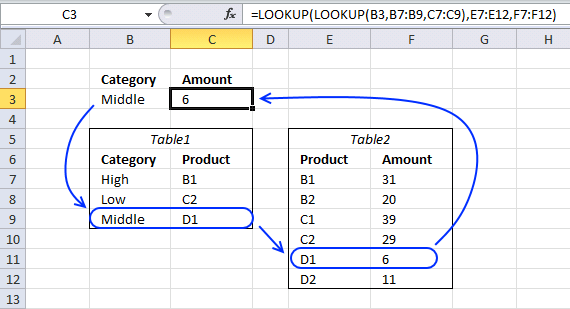This formula looks for a value in table1 and uses the corresponding value to do another lookup in table2. The tables must be related meaning they share at least one column with the same values, in this case column "Product".

Formula in cell C3:

=LOOKUP(LOOKUP(B3, B7:B9, C7:C9), E7:E12, F7:F12)

You can see that the Product column exists in both these tables, the tables are related.

### Explaining formula in cell C3

Note that Table1 is sorted by column B and then by column C, both columns from A to Z.

This is true for Table2 as well, it is sorted by column E and then by column F from A to z.

#### Step 1 - Find value in B7:B9 and return the corresponding value from C7:C9

LOOKUP(B3, B7:B9, C7:C9)

becomes

LOOKUP("Middle", {"High"; "Low"; "Middle"}, {"B1"; "C2"; "D1"})

and returns "D1".

#### Step 2 - Evaluate the second LOOKUP function

LOOKUP(LOOKUP(B3, B7:B9, C7:C9), E7:E12, F7:F12)

becomes

LOOKUP("D1", E7:E12, F7:F12)

becomes

LOOKUP("D1", {"B1"; "B2"; "C1"; "C2"; "D1"; "D2"}, {31; 20; 39; 29; 6; 11})

and returns 6 in cell C3.

I have made more formulas for related tables, see these posts:

## 8. How to lookup multiple values simultaneously (array formula)The first argument lookup_value allows you to enter not only a single value but multiple values. The LOOKUP function returns an array that has the same size as the lookup_value, to demonstrate this I made this formula:

Array formula in cell C3:

=MAX(LOOKUP(B3:B4,B7:B9,C7:C9))

Note that it is required to enter the formula as an array formula if you have an earlier Excel version than Excel 365.

### How to enter an array formula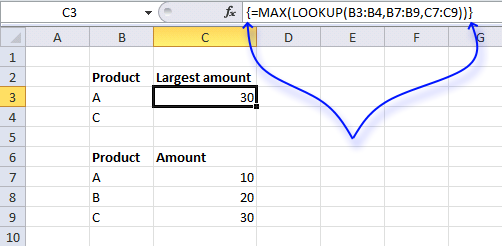1. Select a cell.
2. Copy/Paste the formula to the cell.
3. Press and hold CTRL + SHIFT simultaneously.
4. Press Enter once.
5. Release all keys.

If you did this right the formula begins with a curly bracket { and ends with a curly bracket }. See the formula bar in the picture above.

These characters appear automatically, don't enter these chars yourself.

Excel 365 subscribers don't need to enter this formula as an array formula, simply press Enter.

### Explaining formula in cell C3

Note that the columns must be sorted from A to Z or smallest to largest.

#### Step 1 - Evaluate LOOKUP function

LOOKUP(B3:B4,B7:B9,C7:C9)

becomes LOOKUP({"A";"C"},{"A";"B";"C"},{10;20;30})

and returns this array {10;30}.

#### Step 2 - Extract maximum value from array

The MAX function returns the largest value from a cell range or array.

MAX(LOOKUP(B3:B4,B7:B9,C7:C9))

becomes

MAX({10;30})

and returns 30 in cell C3.